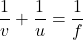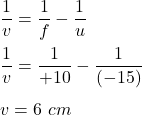## A small object is placed on the principal axis of a convex mirror of focal length 10cm . Determine the position of the image when the object

Question

A small object is placed on the principal axis of a convex mirror of focal length 10cm . Determine the position of the image when the object is 15cm from the mirror.​

in progress 0
5 months 2021-08-27T23:04:16+00:00 1 Answers 0 views 0

v = 6 cm

Explanation:

We have,

A small object is placed on the principal axis of a convex mirror of focal length 10 cm. The focal length of a convex mirror is positive. So, f = +10 cm

It is required to find the position of the image when the object is 15 cm from the mirror.​ Using mirror’s formula :v is image distanceHence, the image will formed at a distance of 6 cm form the mirror.